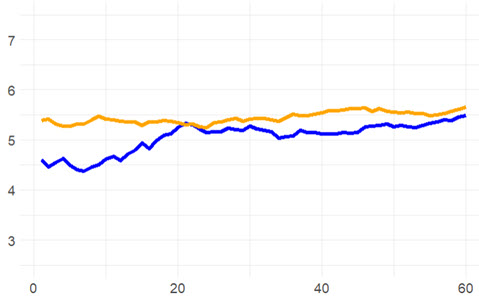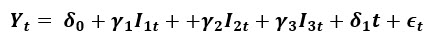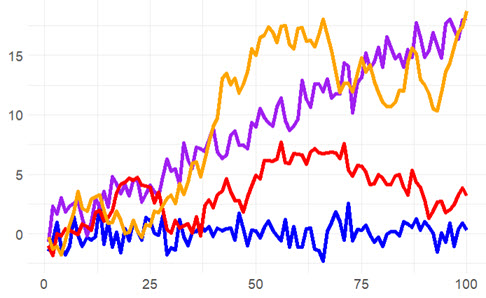# P1.T2.21.2. Unit roots and Dickey-Fuller

#### David Harper CFA FRM

##### David Harper CFA FRM
Staff member
Subscriber
Learning objectives: Explain the challenges of modeling time series containing unit roots. Describe how to test if a time series contains a unit root. Explain how to construct an h-step-ahead point forecast for a time series with seasonality. Calculate the estimated trend value and form an interval forecast for a time series.

Questions:

21.2.1. Peter plotted the last three months (n = 60 days) of two time series: the Russell 1000 index, and the USDJPY exchange rate. His graph is displayed below. Rather than raw values, both series are plotted as log values; i.e., log(index) and log(USDJPY).He perceives a linear relationship and tests this by regressing the index against the exchange rate. The t-statistic is 4.725 (with a p-value of 0.0000150) and the R^2 is 0.278. He decides that the exchange rate is a strong predictor of the index. Before reporting to the Committee, he shows his analysis to his boss, Janice. By writing a single function call, adf.test(), which performs an Augmented Dickey-Fuller test, Janice demonstrates to Peter that both series do have unit roots. Knowing this, Is Peter correct to conclude that the two time series have a relationship?

a. Yes, those values suggest a valid relationship
b. No, because he should not be taking the log; he should regress with raw values
c. No, given the unit roots, he should instead test a regression of differenced data series
d. No, because the R^2 is too low, and this might be expected given the sample size is too small for this purpose

21.2.2. As an input to her firm's research project, Wendy simulates the quarterly growth rate of housing stats with a seasonal model that is plotted below.Because this is a model of deterministic seasonality, she uses seasonal dummy variables. To model the non-zero growth rate, she also includes a term for the linear time trend. In this way, her model has the following form:Her colleague Roy likes the model and he wants to use it in his own time series modeling project. However, his software requires a stationary version of Wendy's series. What can Roy do to transform Wendy's series into a stationary series?

a. The model is already stationary, no transformation is required
b. He only needs a single transformation into the seasonal lag-4 differenced series given by ΔY(t) = Y(t) - Y(t-4)
c. He should add a sufficient number of lag operators so that the series can be expressed as a cubic (aka, third degree) polynomial
d. It is impossible to transform the series into a stationary series because the dummy variables render the mean unstable

21.2.3. Jeremy simulates four time series: white noise, white noise with trend, a random walk, and a random walk with drift. They are plotted below.Jeremy conducts an Augmented Dickey-Fuller (ADF) test with the R function adf.test(). According to GARP, the ADF is the most widely used unit root test. Its null hypothesis is that the series is a unit root process and its corresponding alternative hypothesis is that the series is stationary. Importantly, this adf.test() does allow an intercept and trend: it evaluates a trend-stationary process as stationary but evaluates a difference-stationary process (prior to transformation) as non-stationary.

If Jeremy performs an ADF test on each of these four series, what is the most likely outcome?

a. All four have low p-values; e.g., p-values below 0.050
b. All four have high p-values; e.g., p-values above 0.100
c. The random walks have low p-values but the white noise processes have high p-values
d. The white noise processes have low p-values but the random walks have high p-values